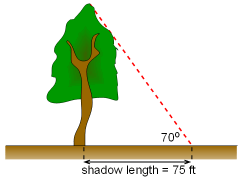Ch 12. Infinite Sequences and Series Multimedia Engineering Math Sequencesand Series Integral andComparison Alt. Series &Abs. Conv. PowerSeries Taylor, Mac. &Binomial
 Chapter 1. Limits 2. Derivatives I 3. Derivatives II 4. Mean Value 5. Curve Sketching 6. Integrals 7. Inverse Functions 8. Integration Tech. 9. Integrate App. 10. Parametric Eqs. 11. Polar Coord. 12. Series Appendix Basic Math Units Search eBooks Dynamics Fluids Math Mechanics Statics Thermodynamics Author(s): Hengzhong Wen Chean Chin Ngo Meirong Huang Kurt Gramoll ©Kurt GramollMATHEMATICS - CASE STUDY IntroductionDiscovery of a Giant Tree by a Botanist A world renowned botanist was exploring Humboldt Redwoods State Park in California. He noticed a giant tree in the forest, and thought it might be the tallest tree known. The shadow of the tree was estimated to be 75 feet and the angle of elevation between the treetop from the tip of the shadow was around 70o. How could he estimate the height of the giant tree using a calculator with only basic mathematics operations (no scientific functions)? Is this the tallest tree? Questions Determine the height of the tree using Taylor series. ApproachProblem Diagram Base the analysis by retaining at least three terms for the series. The tallest redwood recorded is around 370 ft.